Subject: Math

Lesson Length: 30 - 40 mins

Topic: Operations & Algebraic Thinking- Factors pairs/ Prime or Composite Numbers

Standards: CCSS.MATH.CONTENT.4.OA.B.4

Brief Description: In this lesson, students will learn about prime and composite numbers and their factors.

Know Before You Start:

• This lesson is a great introduction to prime and composite numbers.
• Pairs of dice will be needed.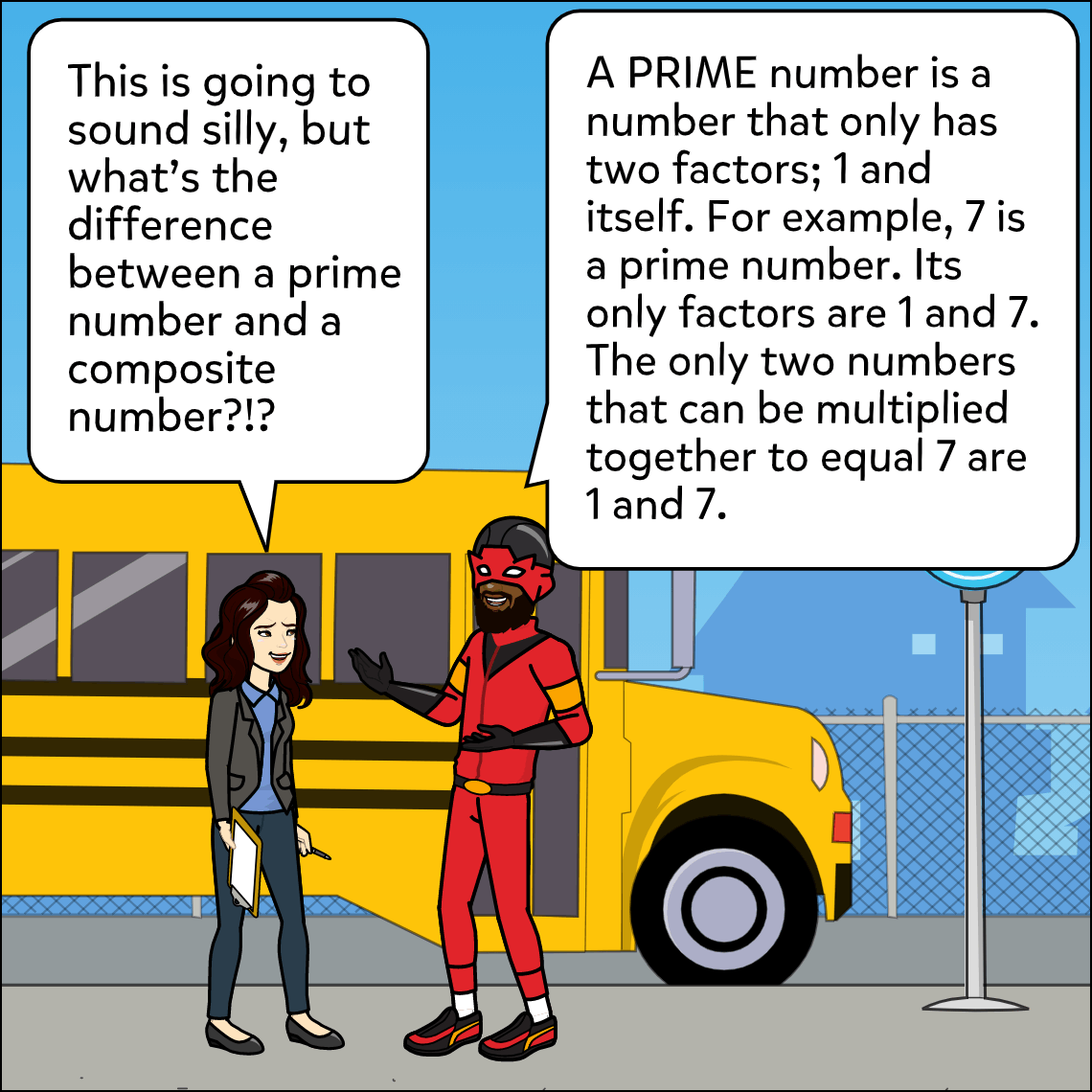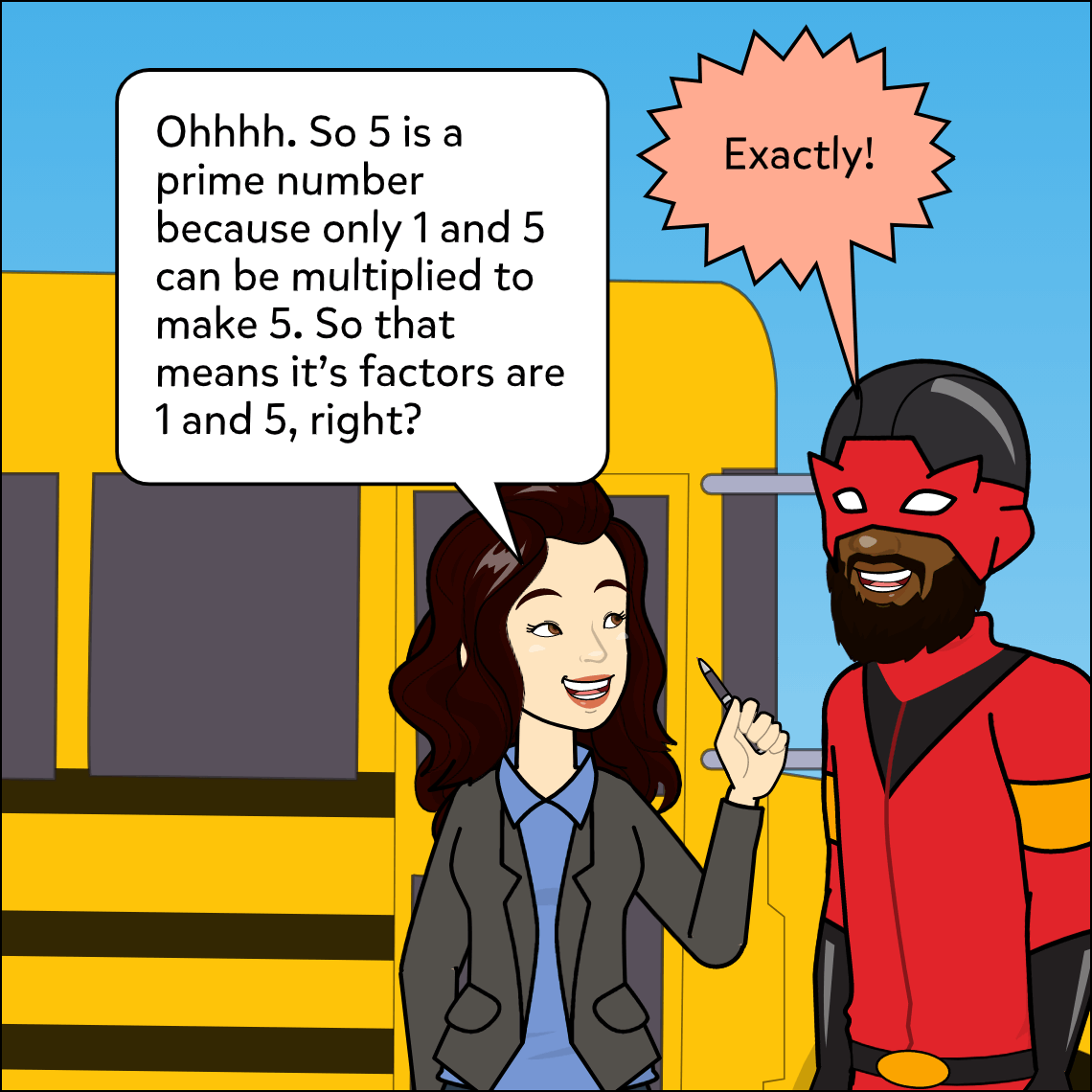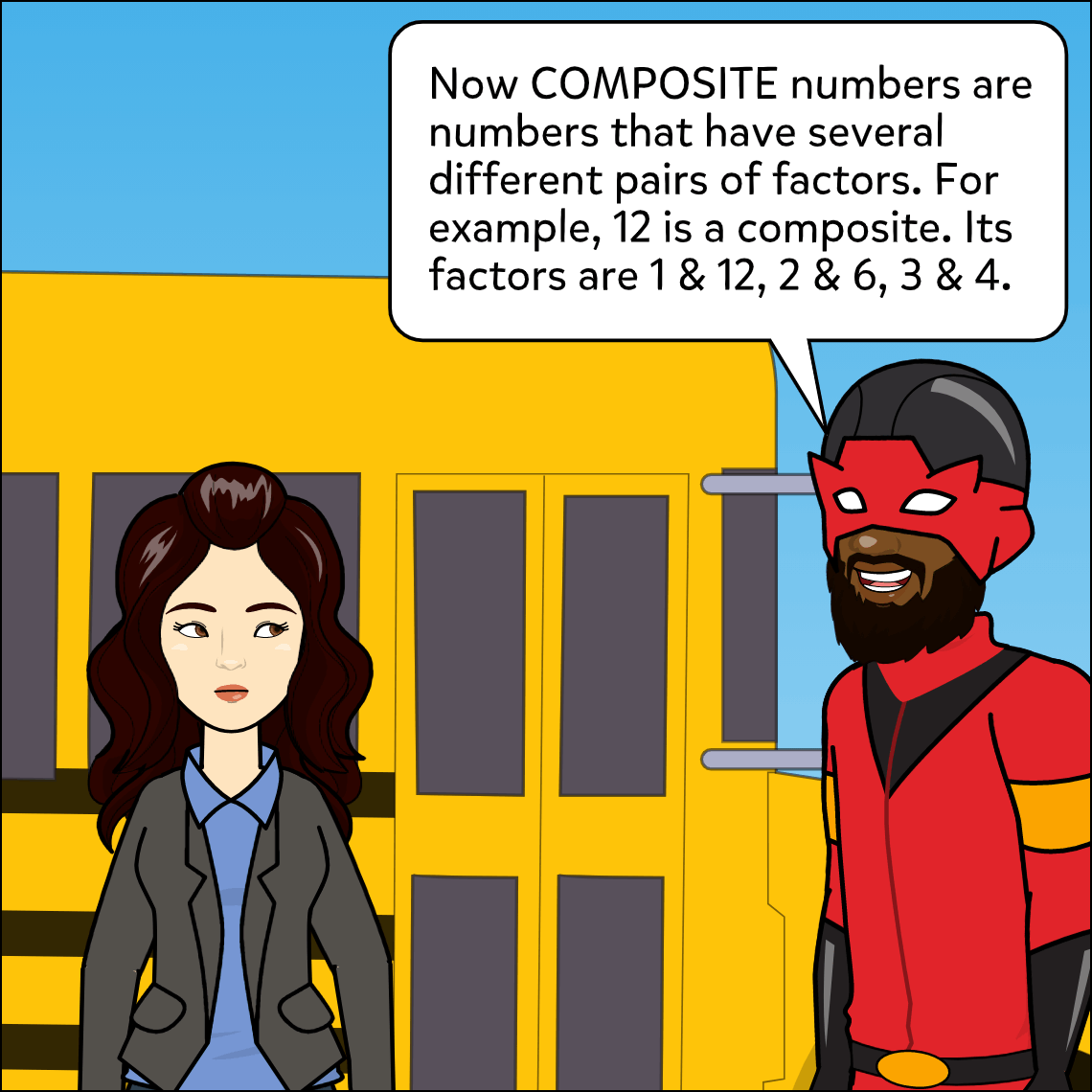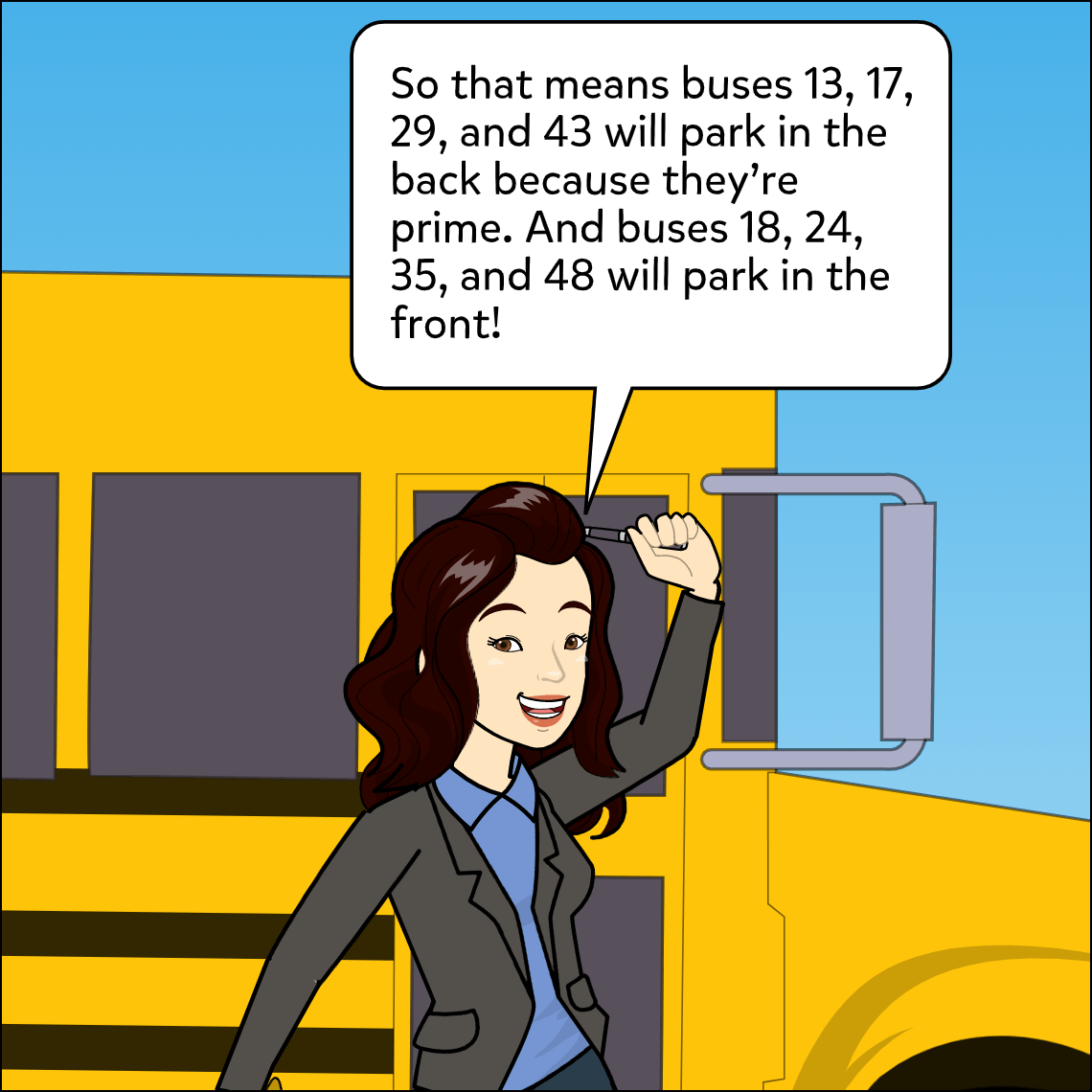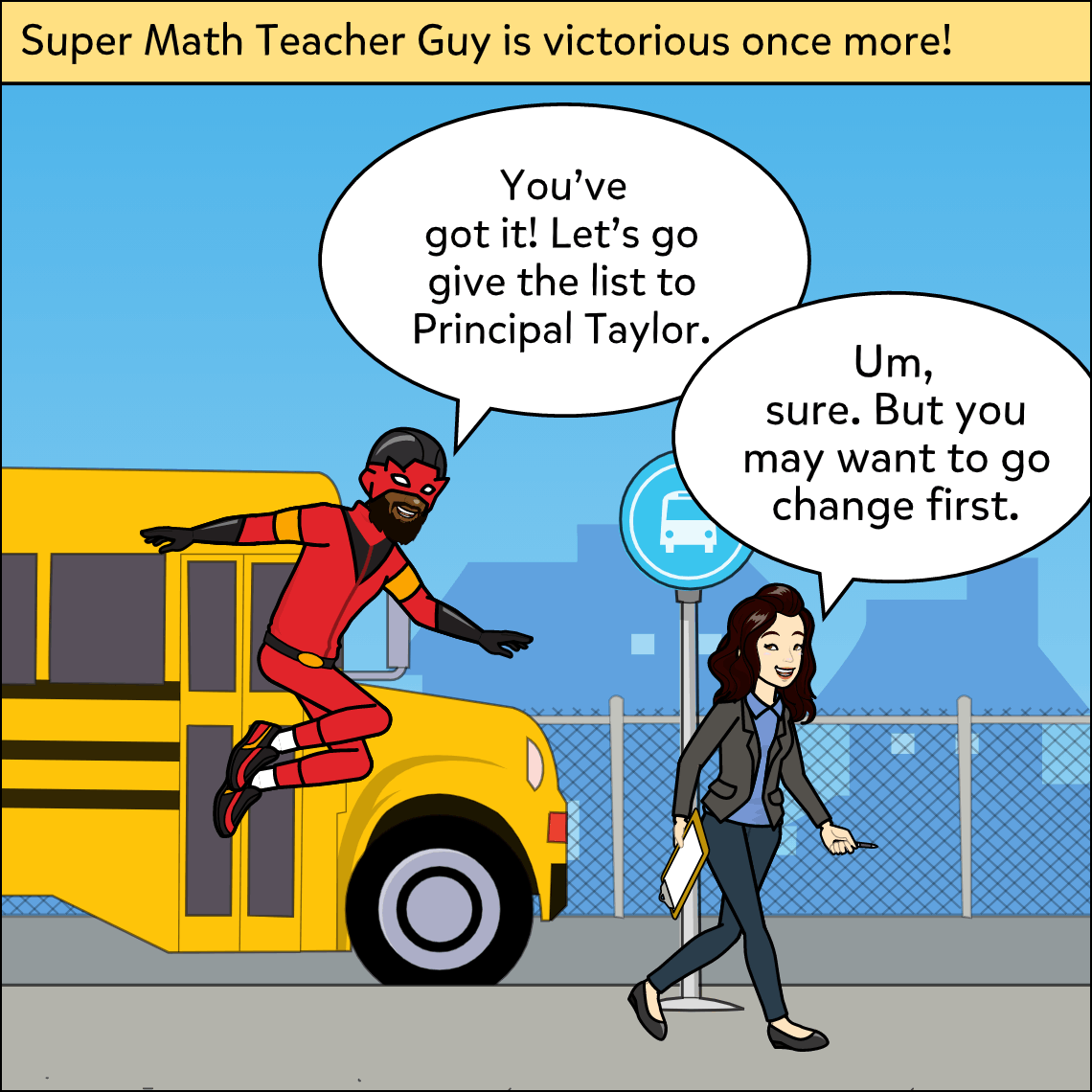Hook:

• Read and discuss the sample comic.  What’s the difference between a prime and composite number? Is Maggie correct about the buses?
• Practice finding the factors of prime and composite numbers as a class.
• Next, have students work in pairs, taking turns providing numbers for their partner to determine the factors of.  Is the number prime or composite?

Activity: It’s time to create Prime and Composite Number Comics.

• Give each student a pair of dice
• Have them roll the dice to create a two digit number (one number from each die)
• Students will now create a 4 frame comic
• In the first frame, students will explain the difference between prime and composite numbers by providing a definition/explanation in their own words.
• In each of the following comic frames, students will share the number they rolled, determine if it is prime or composite and provide the factors.
• Students will repeat this process for 3 separate numbers.

Closure: Have students share their favorite frame with a partner.

Differentiation:

• Provide definition of prime and composite numbers for students.
• Provide a prime and composite number chart.
• Provide sentence stem: ____ is a prime/composite number because _______.
• Allow students to work in pairs.
• Allow students to use speech-to-text feature.

Resources: Comic to print or display: Comic 1.